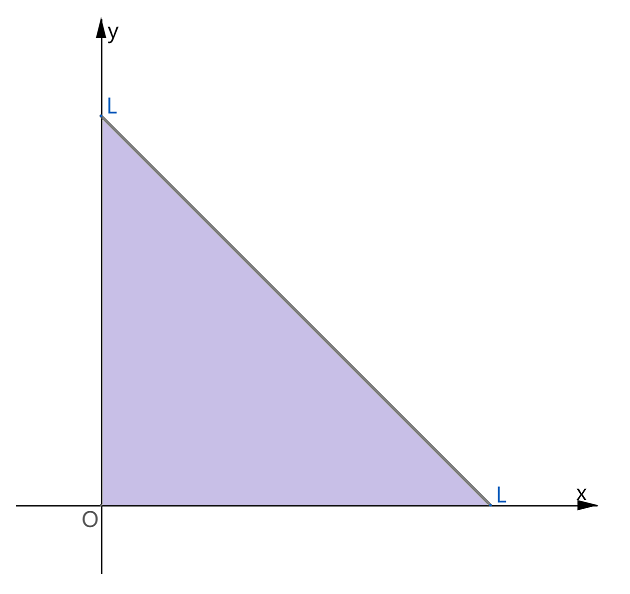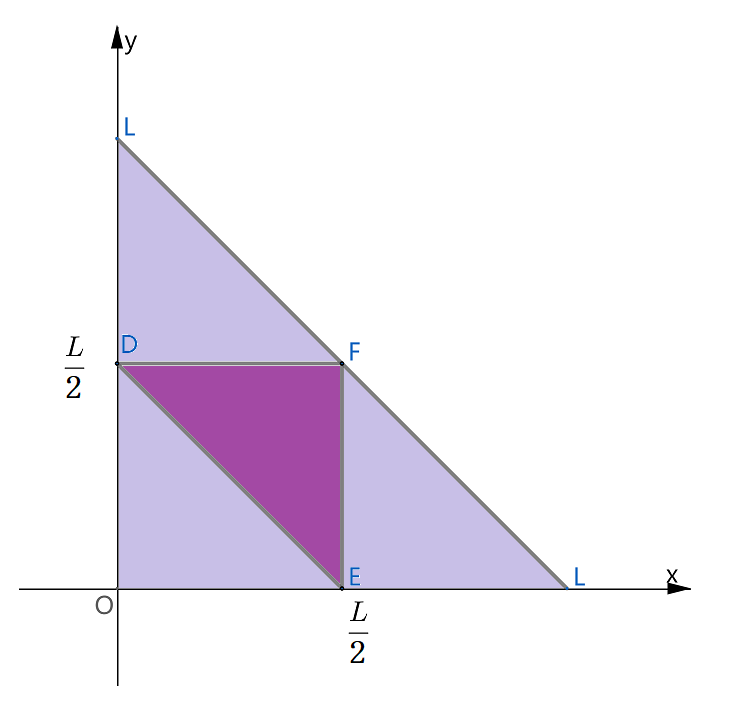安卓手机扫描二维码安装App

[题目]将长为L的一个木棒截为三段，求此三段小木棒能够构成三角形的概率.

[分析]设出所截三段的长度，将它们的取值范围平面上的区域表示出来，将问题转换为与面积有关的几何概型问题.

[]

x和y的全部取值范围Q为：﻿$0﻿ ,﻿$0﻿ , ﻿$0﻿ ，它们在平面上构成下图中的淡紫色区域，即将木棒截成三段的所有可能的 ﻿$(x,y)$﻿ 组合都将落在此淡紫色三角形区域内：﻿$x+y>L-x-y$﻿

﻿$L-x-y+x>y$﻿

﻿$L-x-y+y>x$﻿﻿$P(M)=$﻿﻿$\dfrac{\text{M的面积}}{\text{Q的面积}}$﻿ ﻿$=?$﻿

A. ﻿$\dfrac{1}{2}$﻿ B. ﻿$\dfrac{1}{3}$﻿

C. ﻿$\dfrac{1}{4}$﻿ D. ﻿$\dfrac{3}{5}$﻿苹果手机扫描二维码安装App